# CenterNet Trained onMS-COCO Data

Detect and localize objects in an image

Released in 2019, this family of object detection models detects objects by their central point instead of directly computing axis-aligned boxes. The models exploit keypoint estimation techniques to find center point locations from generated heat maps and then regress the box sizes and offsets. The center locations are predicted per class, while box sizes and offsets are class agnostic. Compared to the anchor-based approaches, CenterNet does not suffer from the extremely large amounts of box candidates that require complicated labeling methods as well as additional post-processing like non-maximum suppression. Also, the detector does not make any implicit assumptions on the objects scales and aspect ratios, contrary to the other popular approaches that encode them in the anchors.

## Training Set Information

• Microsoft COCO, a dataset for image recognition, segmentation and captioning, consisting of more than three hundred thousand images in 80 different object classes.

## Model Information

•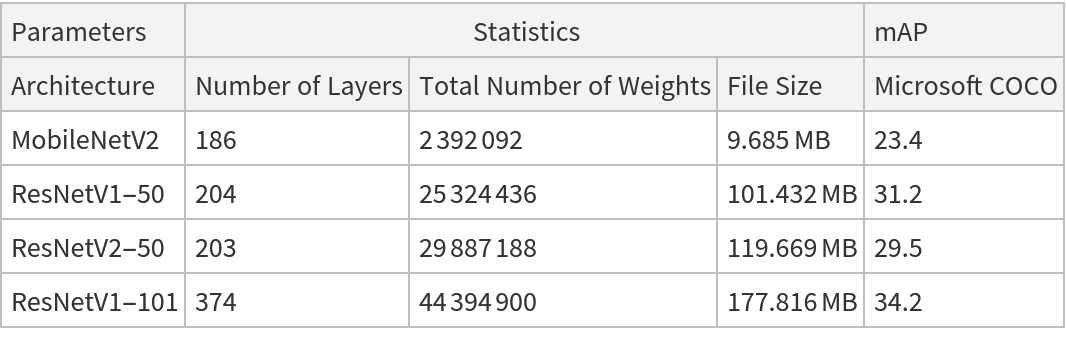## Examples

### Resource retrieval

Get the pre-trained net:

 In:=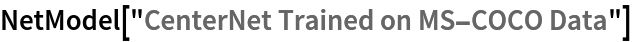Out=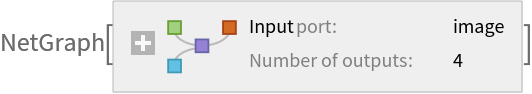### NetModel parameters

This model consists of a family of individual nets, each identified by a specific parameter combination. Inspect the available parameters:

 In:=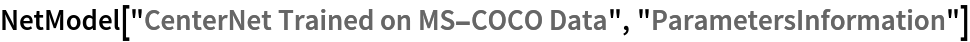Out=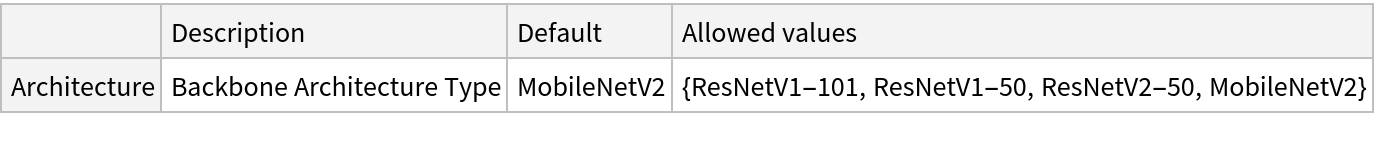Pick a non-default net by specifying the parameters:

 In:=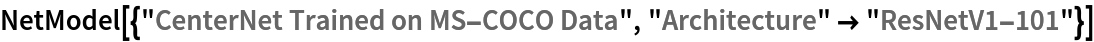Out=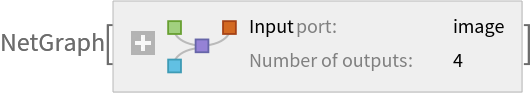Pick a non-default uninitialized net:

 In:=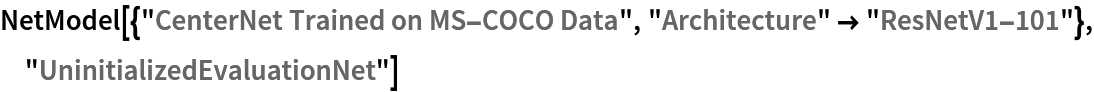Out=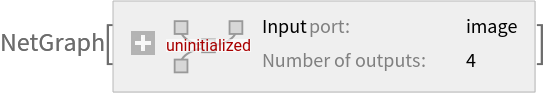### Evaluation function

Define the label list for this model:

 In:=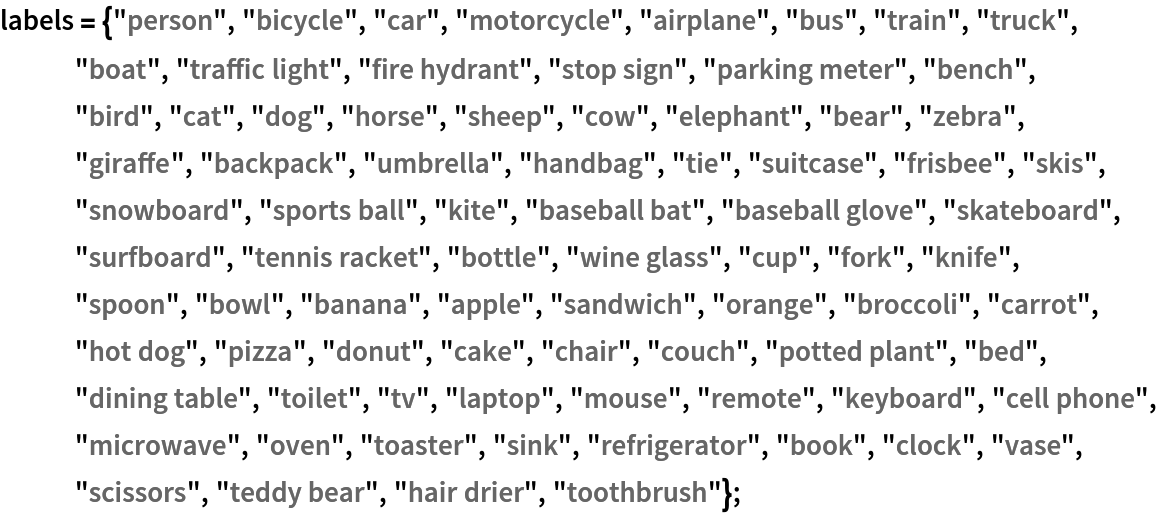Write an evaluation function to scale the result to the input image size and suppress the least probable detections:

 In:=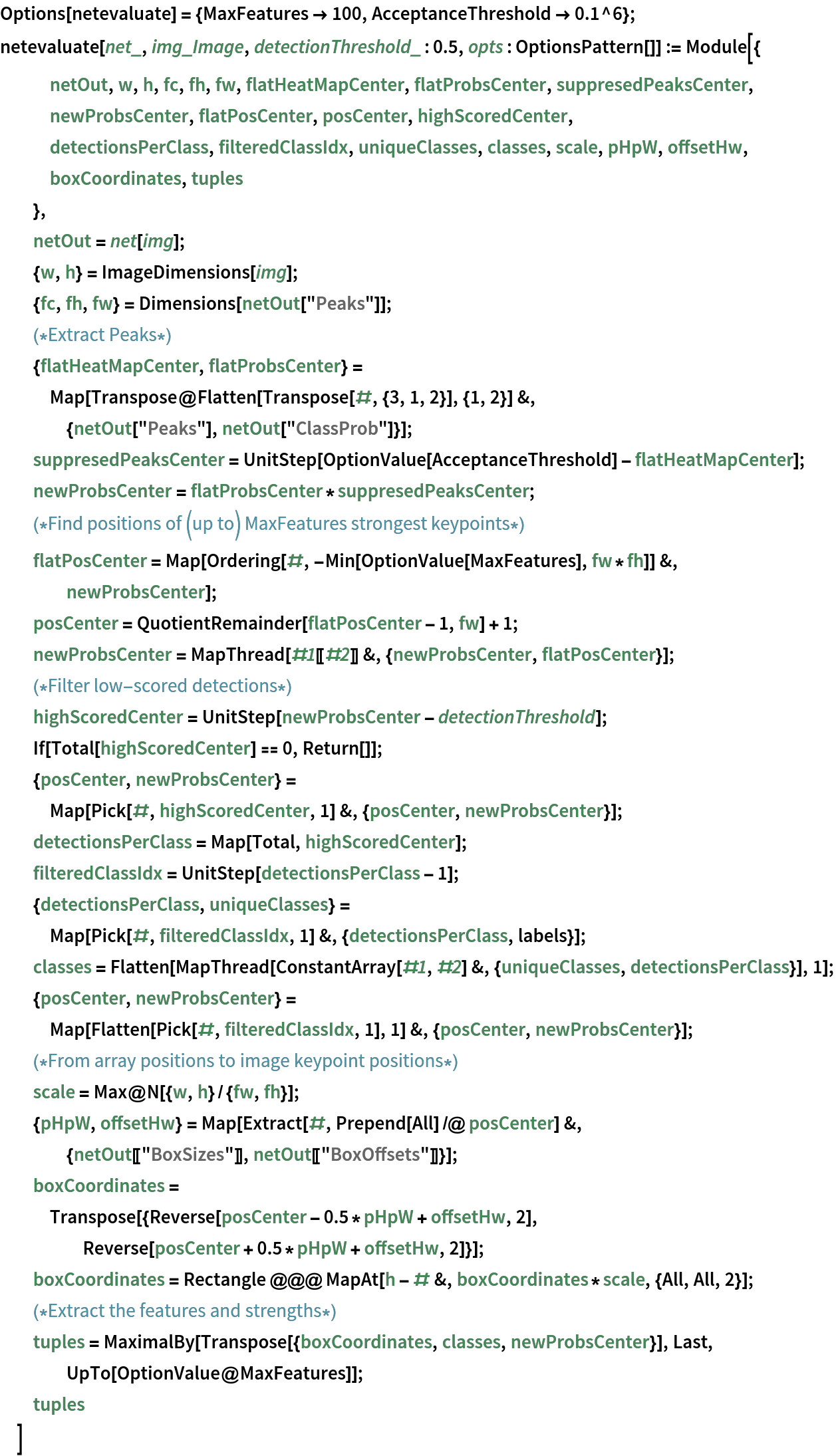### Basic usage

Obtain the detected bounding boxes with their corresponding classes and confidences for a given image:

 In:=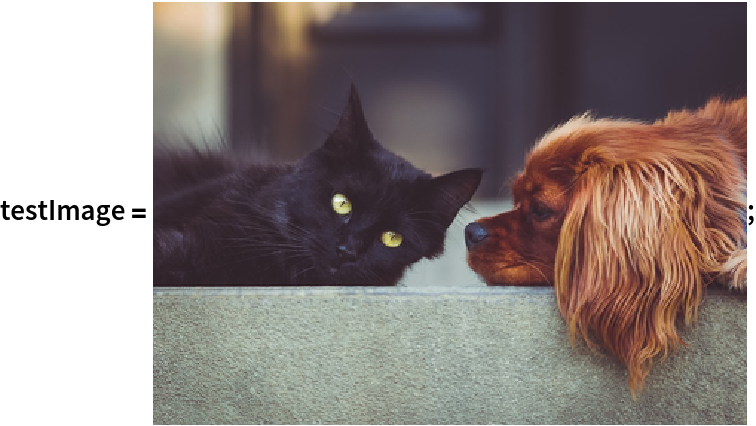In:=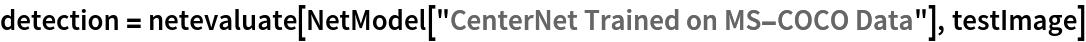Out=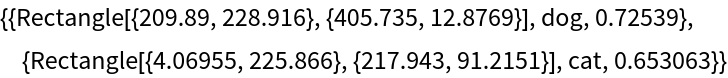Inspect which classes are detected:

 In:=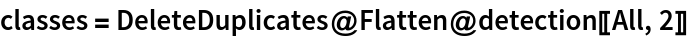Out=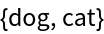Visualize the detection:

 In:=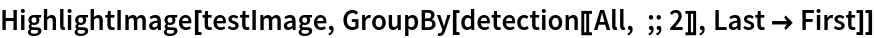Out=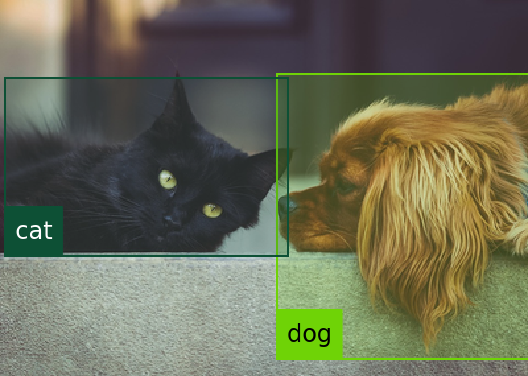### Network result

For the default input size of 512x512, the net produces 128x128 bounding boxes whose centers mostly follow a square grid. For each bounding box, the net produces the box’s size and the offset of the box’s center with respect to the square grid:

 In:=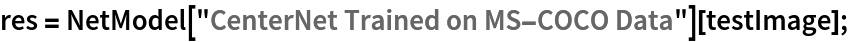In:=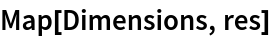Out=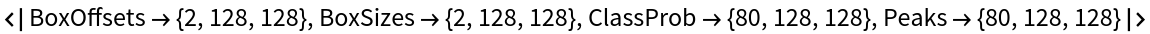Change coordinate system into a graphics domain:

 In:=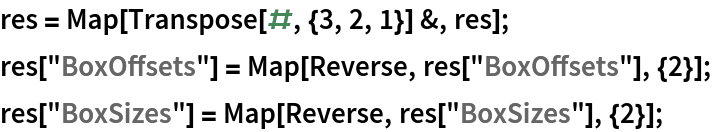Compute and visualize the box center positions:

 In:=Visualize the box center positions. They follow a square grid with offsets:

 In:=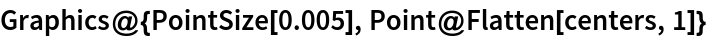Out=Compute the boxes coordinates:

 In:=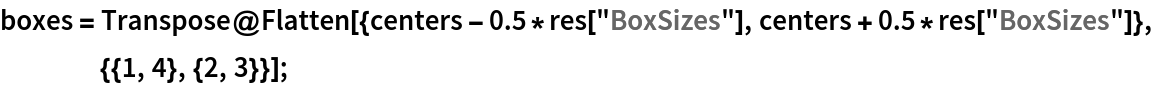In:=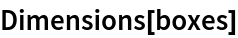Out=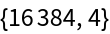Define a function to rescale the box coordinates to the original image size:

 In:=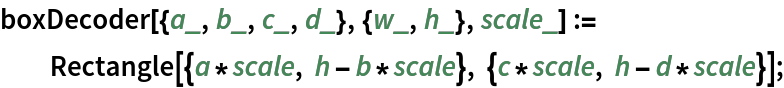Visualize all the boxes predicted by the net scaled by their "objectness" measures:

 In:=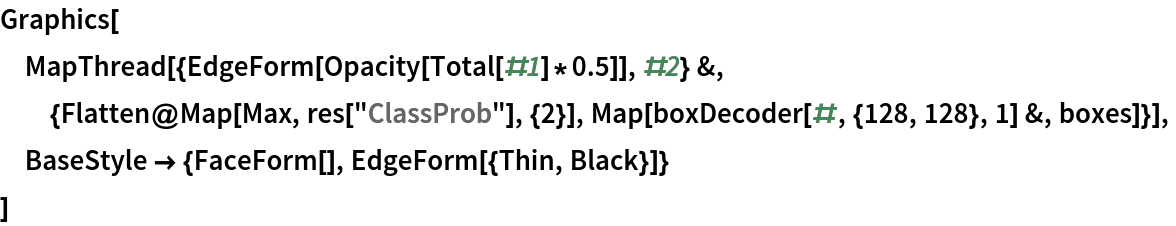Out=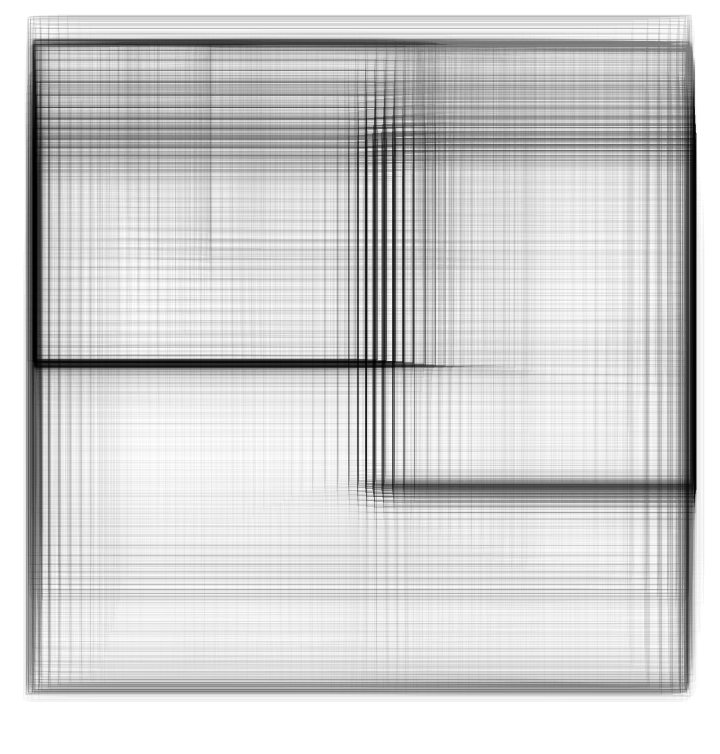Visualize all the boxes scaled by the probability that they contain a cat:

 In:=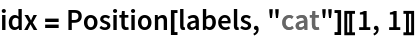Out=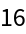In:=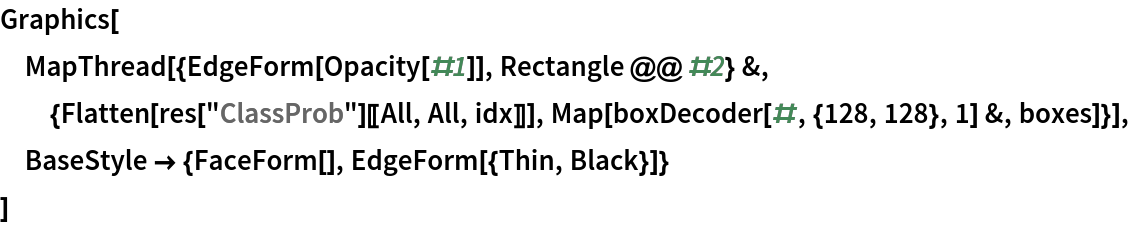Out=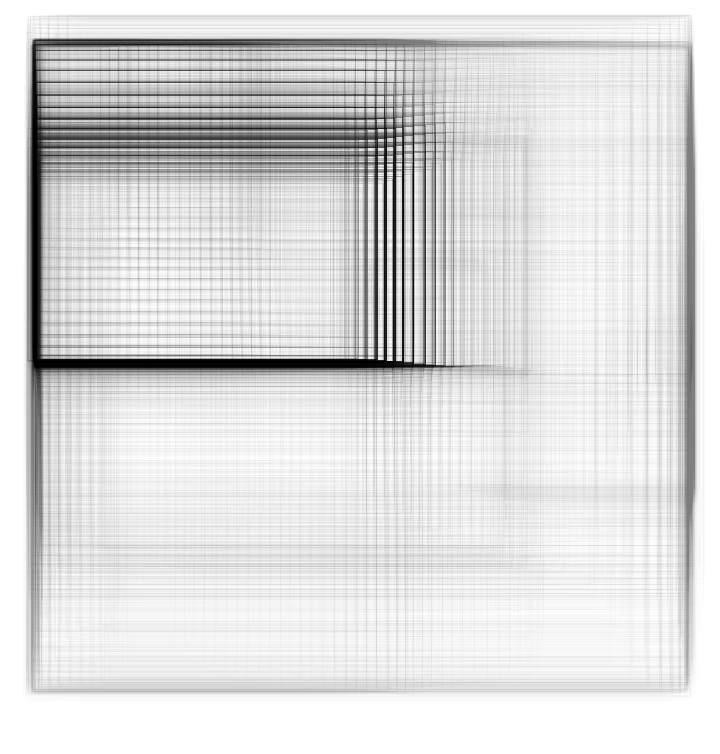Superimpose the cat prediction on top of the scaled input received by the net:

 In:=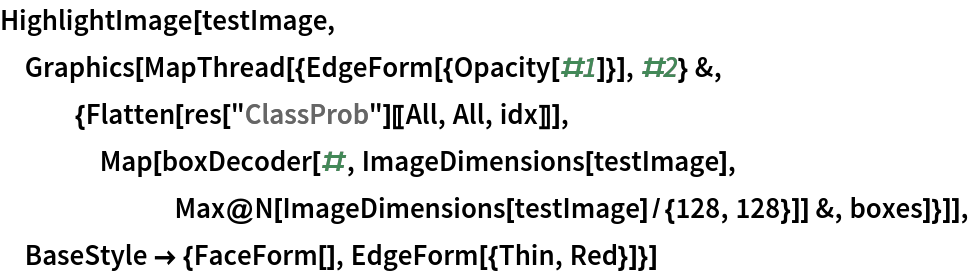Out=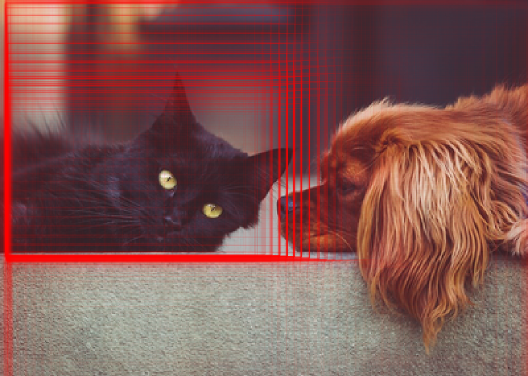### Heat map visualization

Every box is associated to a scalar strength value indicating the likelihood that the patch contains an object:

 In:=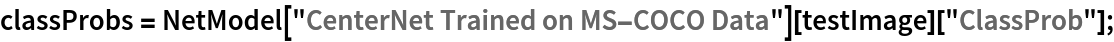In:=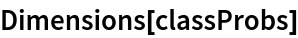Out=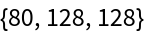The strength of each patch is the maximal element aggregated across all classes. Obtain the strength of each patch:

 In:=Out=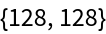Visualize the strength of each patch as a heat map:

 In:=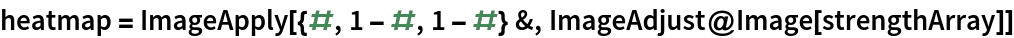Out=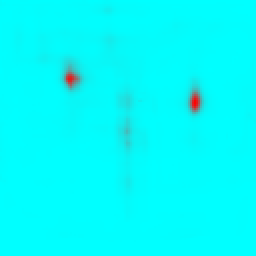Stretch and unpad the heat map to the original image domain:

 In:=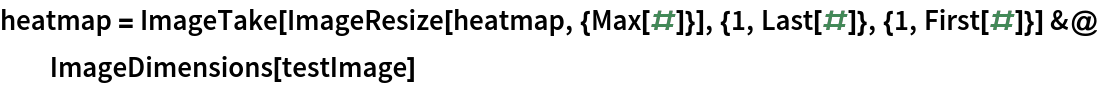Out=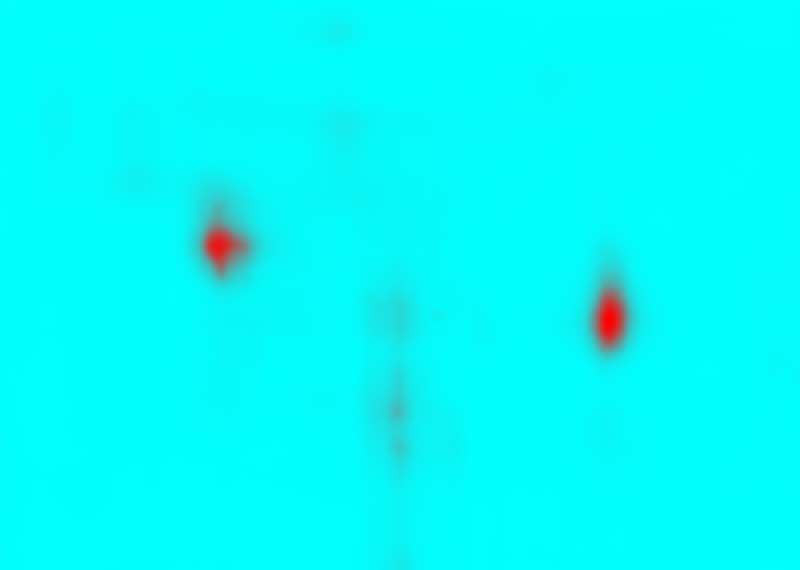Overlay the heat map on the image:

 In:=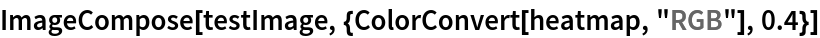Out=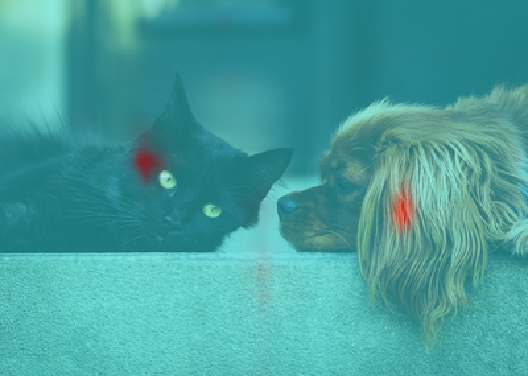Obtain and visualize the strength of each patch for the "cat" class:

 In:=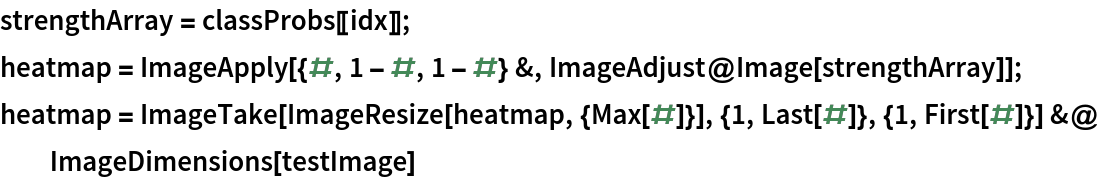Out=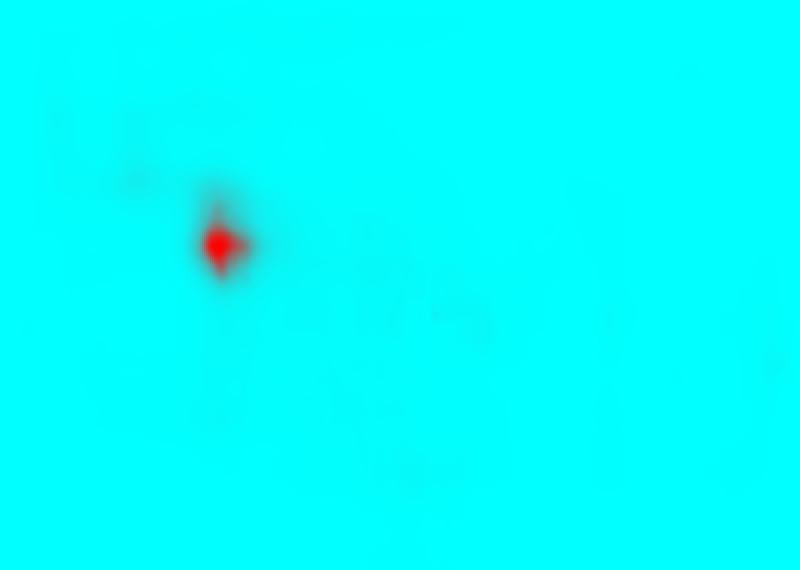Overlay the heat map on the image:

 In:=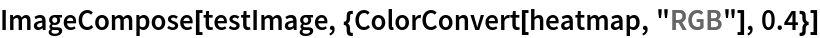Out=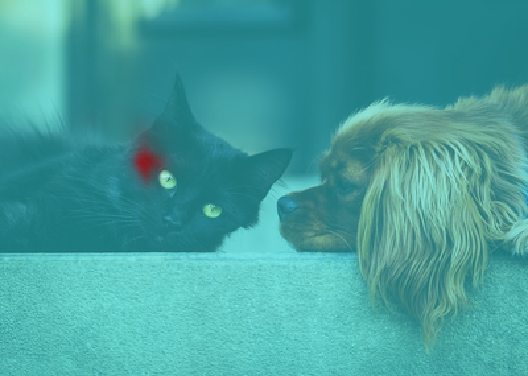Automatic image resizing can be avoided by replacing the NetEncoder. First get the NetEncoder:

 In:=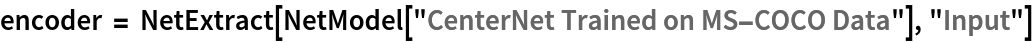Out=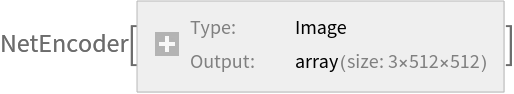Note that the NetEncoder resizes the image by keeping the aspect ratio and then pads the result to have a fixed shape of 512x512. Visualize the output of NetEncoder adjusting for brightness:

 In:=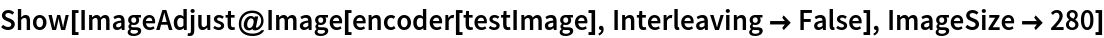Out=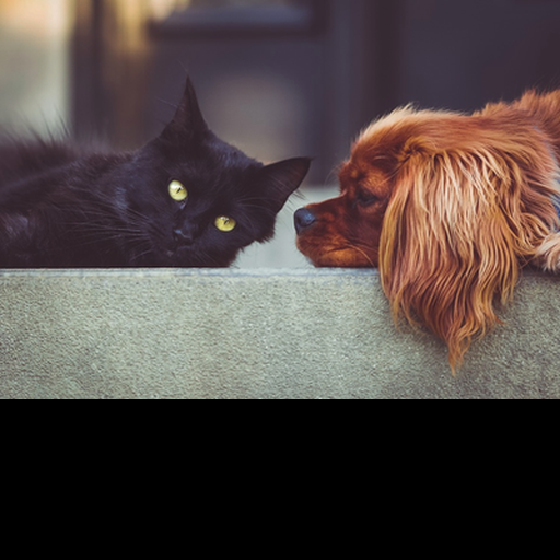Create a new NetEncoder with the desired dimensions:

 In:=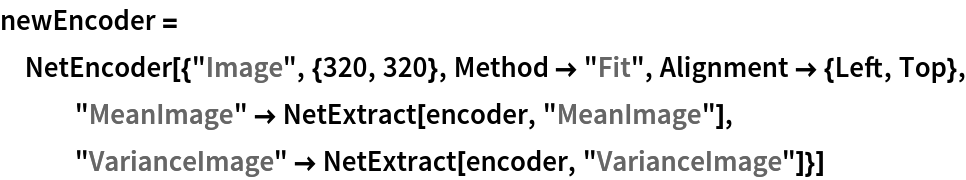Out=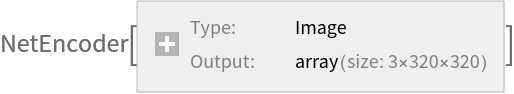Attach the new NetEncoder:

 In:=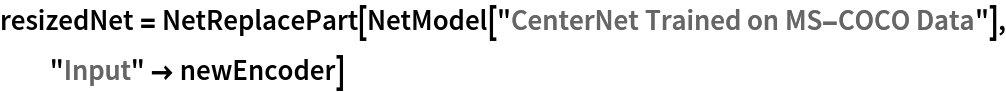Out=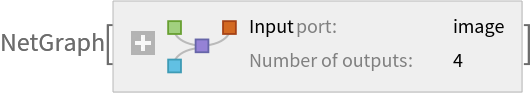Obtain the detected bounding boxes with their corresponding classes and confidences for a given image:

 In:=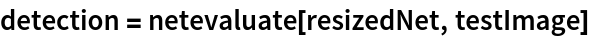Out=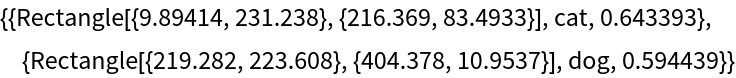Visualize the detection:

 In:=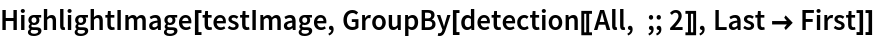Out=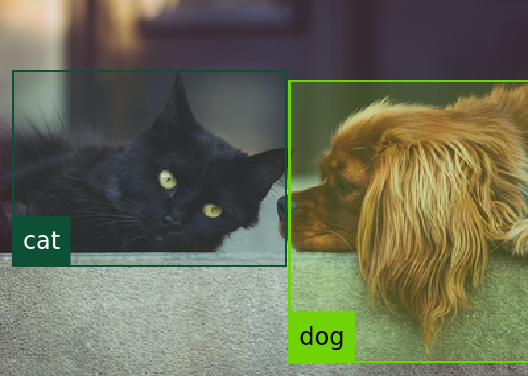Note that even though the localization results and the box confidences are slightly worse compared to the original net, the resized network runs significantly faster:

 In:=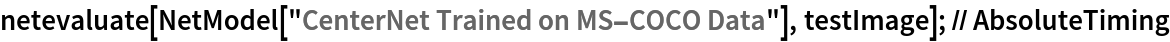Out=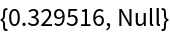In:=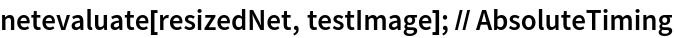Out=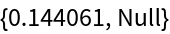### Net information

Inspect the number of parameters of all arrays in the net:

 In:=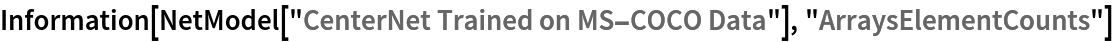Out=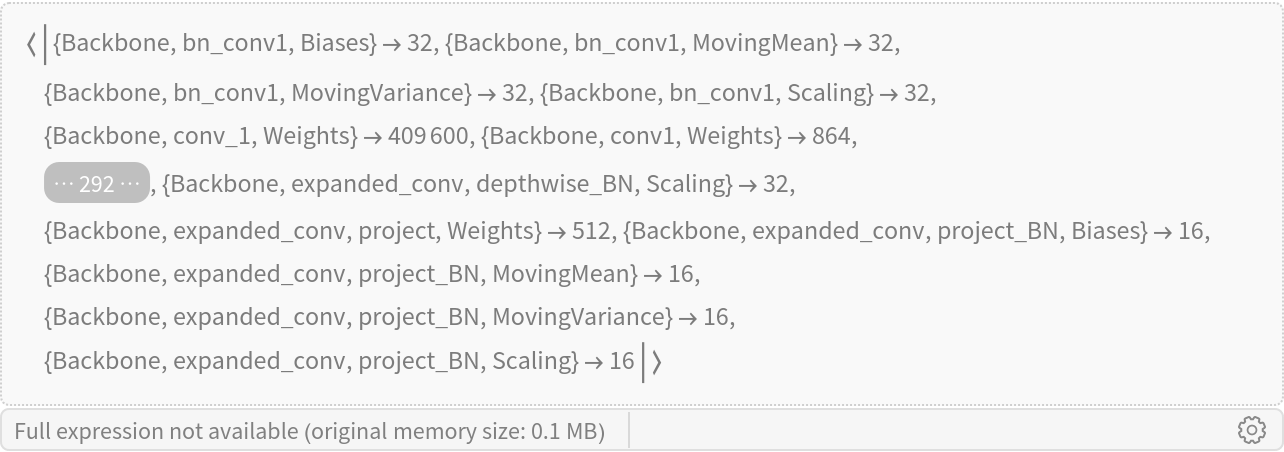Obtain the total number of parameters:

 In:=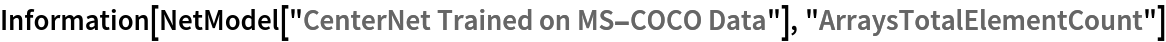Out=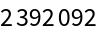Obtain the layer type counts:

 In:=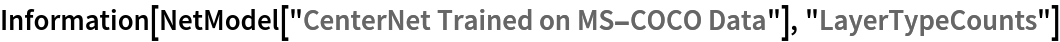Out=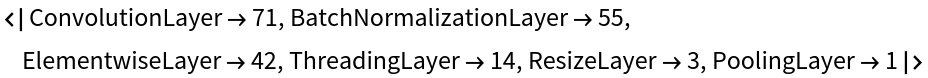Display the summary graphic:

 In:=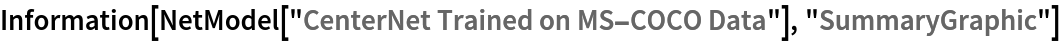Out=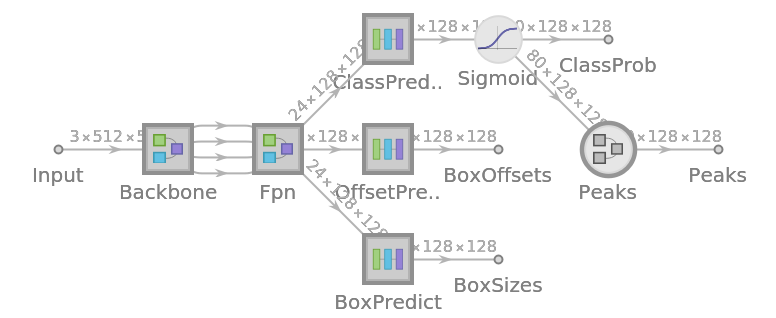## Reference

• X. Zhou, D. Wang, P. Krähenbühl, "Objects as Points," arXiv:1904.07850 (2019)